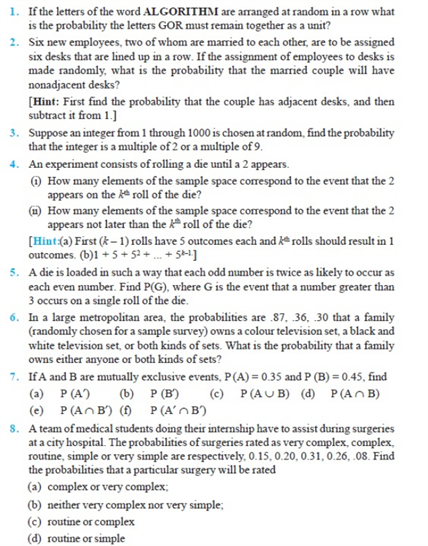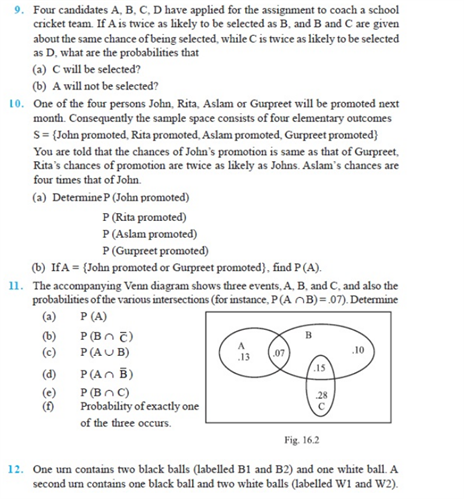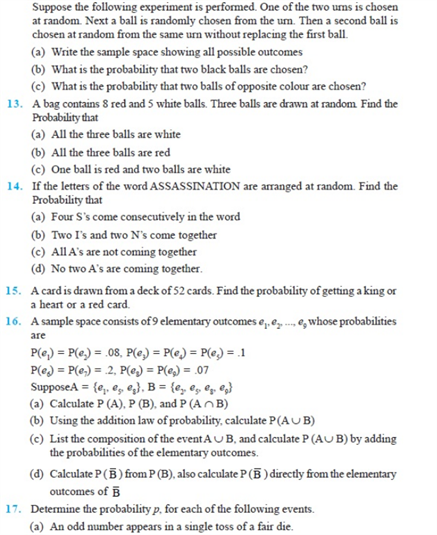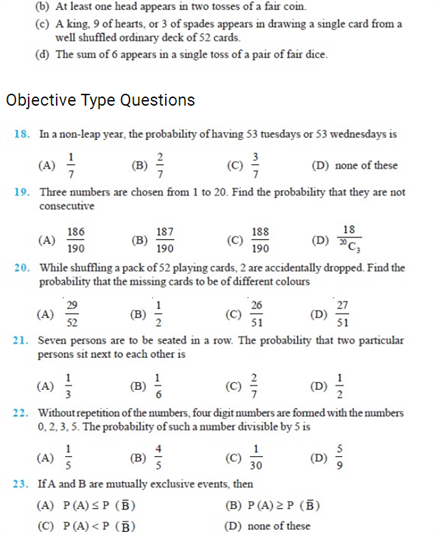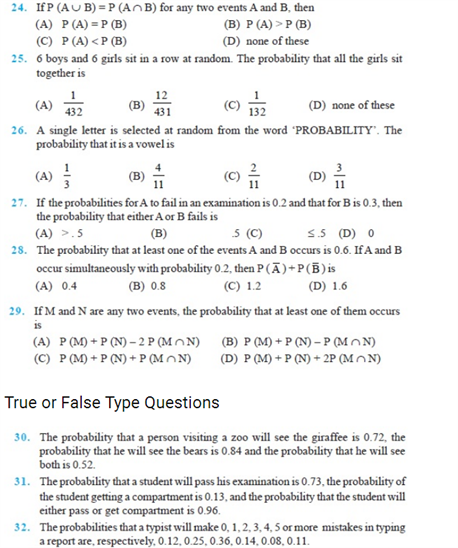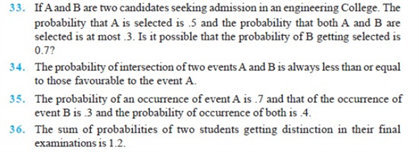# Chapter 16 - Probability

Some of the most important Probability Class 11 questions i.e chapter 16 are given here. These chapter 16 class 11 maths questions cover several short answer type questions, long answer type questions and HOTS questions that are crucial for CBSE class 11 exams. Here, some of the important Probability questions class 11 from NCERT are also included.

Also, Check:

Probability and Statistics

Chance and Probability

Events in Probability

## Probability Important Questions For Class 11 (Chapter 16)

These class 11 Probability questions are categorized into short answer type questions and long answer type questions. These questions cover various concepts which will help class 11 students to develop problem-solving skills for the exam.

1. List the sample space is throwing a die? Solution: S = { 1, 2, ,3 ,4 , 5, 6}
2. List the sample space in tossing a coin? Solution: s = { H, T}
3. List the space in simultaneous toss of a die and a coin? Solution: S= {(1,H)   (2,H) (3, H) (4,H)    (5,H) ( 6,H) (1,T) (2,T)    (3,T) (4,T) (5,T) (6,T)}
4. In a single throw of two dice, find the probability of obtaining a total of 8.
5. A bag contains 9 red and 12 white balls. One ball is drawn at random. Find the probability that the ball is drawn is red. (Answer: 3/7)
6. What is the probability that the ordinary year has 53 Sundays? (Answer: 2/7)
7. A card is drawn at random from a well-shuffled pack of 52 cards. Find the probability of its being a spade or a king. (Answer: 4/13)
8.  A card is drawn from a deck of 52 cards. Find the probability of getting a king or a heart or a red card? (Answer: 7/13).
9. A number is chosen from the number 1 to 100. Find the probability of its being divisible by 4 or 6.(Answer: 33/100)
10. Two cards are drawn at random from a pack of 52 cards. What is the probability that both the drawn cards are aces? (Answer: 1/221)
11. A coin is tossed once. Find the probability of getting a tail. (Answer: ½)

### NCERT Class 11 Probability Questions